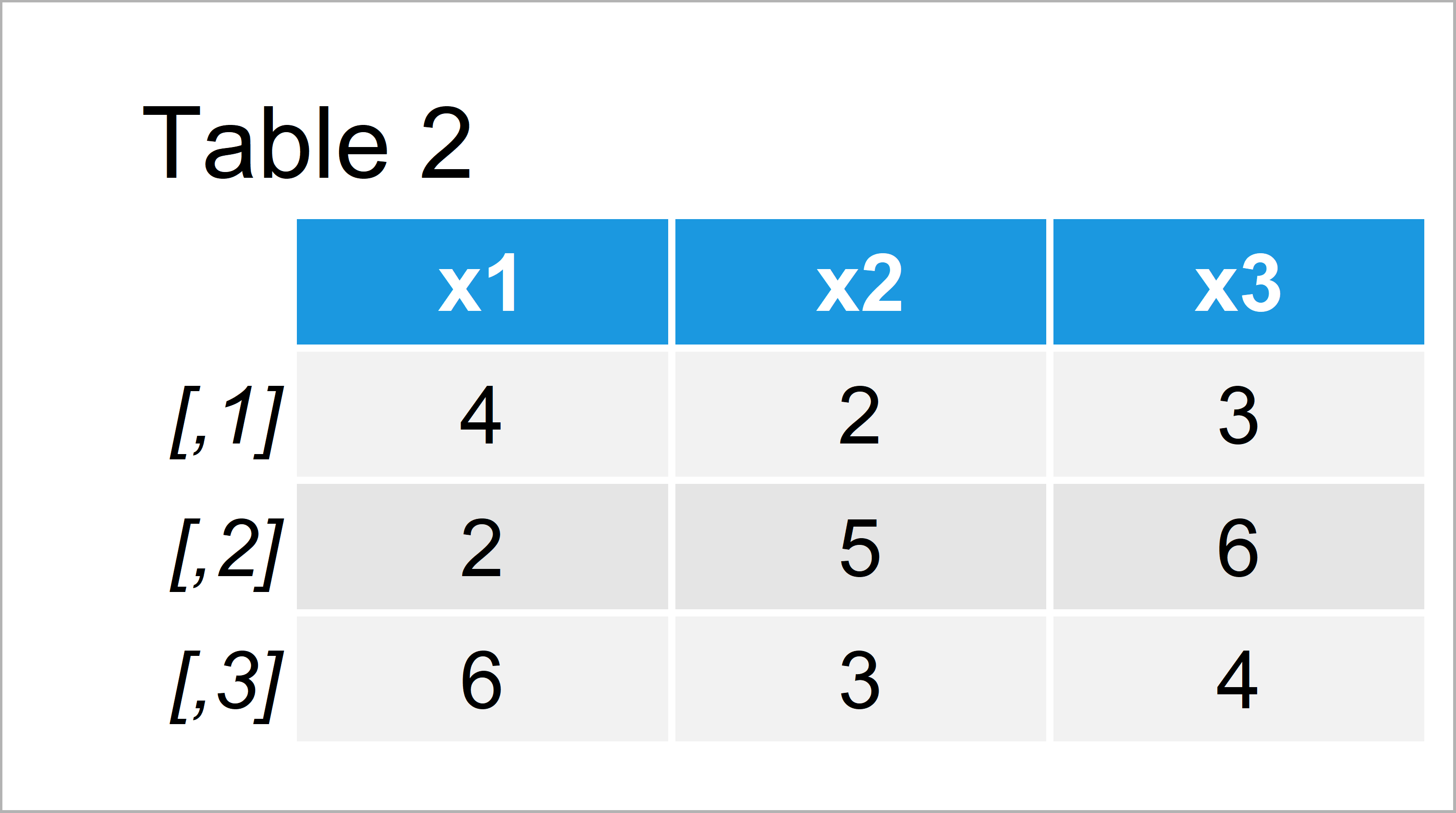# Find Max & Min Length of Character Strings in Columns in R (4 Examples)

In this article, I’ll show how to get the number of characters in in each string data frame variables in the R programming language.

Let’s start right away.

## Creation of Example Data

The first step is to create some data that we can use in the examples below:

```data <- data.frame(x1 = c("Lala", "Lo", "Lelele"),  # Create example data
x2 = c("Hi", "Hello", "Hey"),
x3 = c("aaa", "bbbbbb", "cccc"))
data                                                # Print example data```Have a look at the previous table. It shows that our example data is composed of three rows and three columns. All columns contain character strings with different length.

## Example 1: Get Length of Character Strings in One Variable Using nchar() Function

The following R programming code shows how to get the number of characters in each character string of one specific data frame variable.

For this, we can use the nchar function as shown below:

```nchar(data\$x1)                                      # Length of strings in one column
#  4 2 6```

The first character string in the variable x1 contains four characters, the second string contains two characters, and the third string contains six characters.

## Example 2: Get Length of Character Strings in All Variables Using apply() & nchar() Functions

Example 2 shows how to get the string length of each data frame cell by using the apply and nchar functions.

`apply(data, 2, nchar)                               # Length of strings in all columns`As shown in Table 2, we have created a matrix showing all character string counts by executing the previous R syntax.

## Example 3: Get Maximum & Minimum Length of Character Strings in One Variable Using max(), min() & nchar() Functions

The following syntax explains how to return only the maximum character string count in one specific column:

```max(nchar(data\$x1))                                 # Maximum length of string in one column
#  6```

Of course, we can also apply other functions instead of max. Below, we return the minimum character string length in the column x1:

```min(nchar(data\$x1))                                 # Minimum length of string in one column
#  2```

## Example 4: Get Maximum & Minimum Length of Character Strings in All Variables Using apply(), max(), min() & nchar() Functions

In this example, I’ll show how to return the max and min counting values in each column.

We can extract the maximum number of characters in each column like this…

```apply(data, 2, function(x) max(nchar(x)))           # Maximum length of string in all columns
# x1 x2 x3
#  6  5  6```

…and the minimum number of characters in each column like this:

```apply(data, 2, function(x) min(nchar(x)))           # Minimum length of string in all columns
# x1 x2 x3
#  2  2  3```

Great!

## Video & Further Resources

Have a look at the following video of my YouTube channel. In the video, I’m explaining the R programming syntax of this tutorial:

Please accept YouTube cookies to play this video. By accepting you will be accessing content from YouTube, a service provided by an external third party.If you accept this notice, your choice will be saved and the page will refresh.

Furthermore, you could have a look at the other articles of this website:

To summarize: In this tutorial, I have explained how to return the number of characters in a string in a data frame variable in R. In case you have further questions and/or comments, let me know in the comments section. In addition, please subscribe to my email newsletter in order to receive updates on new tutorials.

Subscribe to the Statistics Globe Newsletter